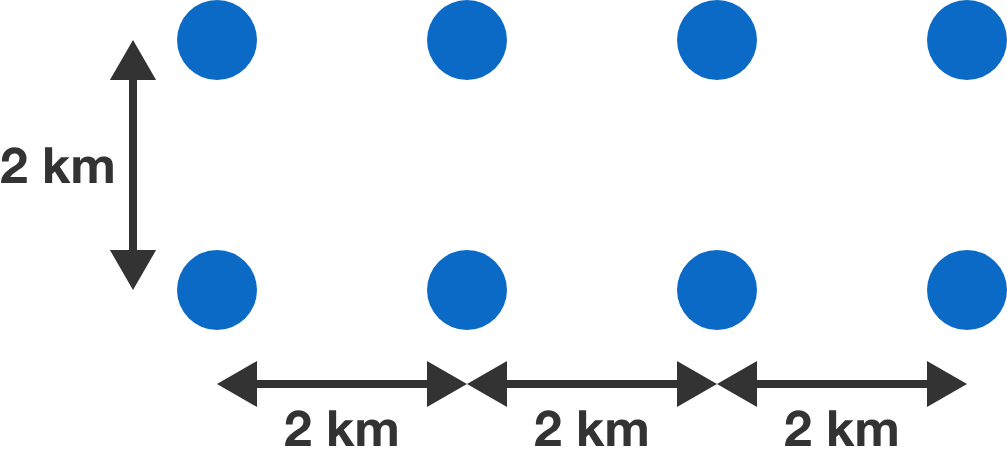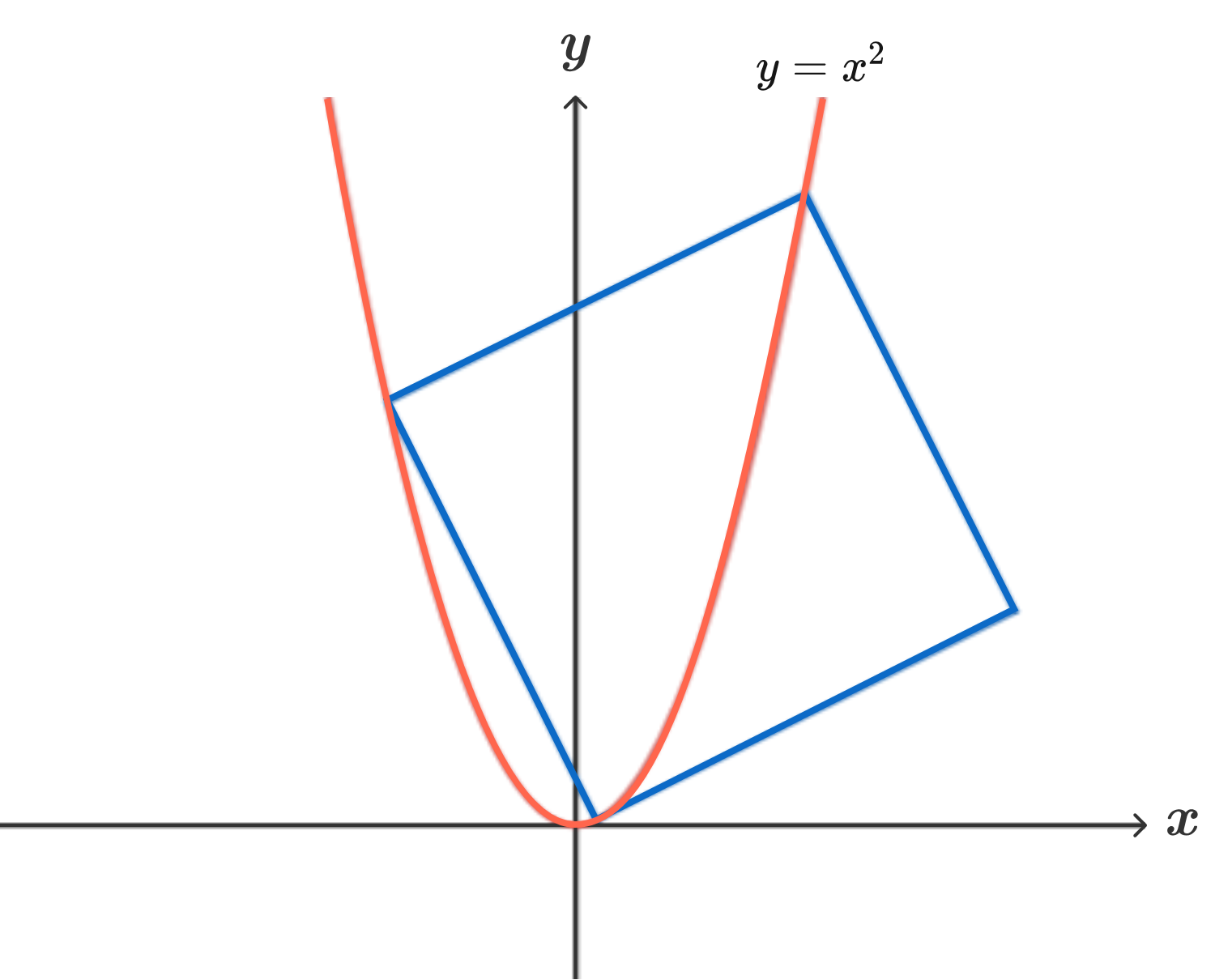# Problems of the Week

Contribute a problem

Mr. Dlanod, a notorious developer, plans to build eight hotels in the wilderness (complete with casinos and golf courses), at the points shown in the diagram. A network of roads needs to be built so that any hotel is connected to any other, but the budget allows for at most 13 km of roads. (We are allowed to introduce crossroads and intersections, of course.)Bonus: What is the minimum total length of such a network of roads?

What is the minimum possible area of a square that has three of its vertices on the parabola $y = x^2$ and its fourth vertex outside of the parabola?Bonus: Find the golden ratio along the way.

For a rational number $x$ that equals $\frac ab$ in lowest terms, let $f(x)=ab.$

What is the value of $\large \sum_{x\in\mathbb Q^+}\frac 1{f(x)^2},$ where the sum extends over all positive rationals?

Bonus: More generally, exhibit an infinite sequence of distinct rational exponents $s$ such that $\displaystyle \sum_{x\in\mathbb Q^+}f(x)^{-s}$ is rational.

What is the smallest positive integer $N$ that can be written as a sum $N=x^2+y^2$ in exactly 11 ways, where $x$ and $y$ are integers with $x \geq y >0?$

How many polynomials $P$ of degree 4 with real coefficients satisfy $P\big(x^2\big)=P(x)P(-x)?$

×